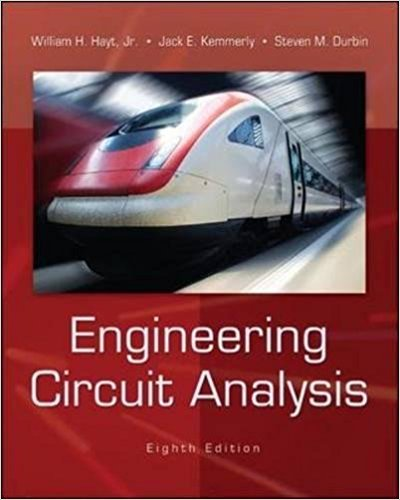×
Get Full Access to Engineering Circuit Analysis - 8 Edition - Chapter 7.1 - Problem 7.1
Get Full Access to Engineering Circuit Analysis - 8 Edition - Chapter 7.1 - Problem 7.1

×

# Determine the current flowing through a 5 mF capacitor in response to a voltage v equalISBN: 9780073529578 304

## Solution for problem 7.1 Chapter 7.1

Engineering Circuit Analysis | 8th Edition

• Textbook Solutions
• 2901 Step-by-step solutions solved by professors and subject experts
• Get 24/7 help from StudySoup virtual teaching assistantsEngineering Circuit Analysis | 8th Edition

4 5 1 350 Reviews
17
2
Problem 7.1

Determine the current flowing through a 5 mF capacitor in response to a voltage v equal to: (a) 20 V; (b) 2e5t V.

Step-by-Step Solution:
Step 1 of 3

Lecture 6: Dimensions and Units Outcomes  Explain the difference between units and dimensions  List the base (fundamental) dimensions in the SI system  Identify derived dimensions and units  Perform dimensional homogeneity checks on equations  Explain the concept and use of dimensionless numbers  Use common prefixes and prefix symbols...

Step 2 of 3

Step 3 of 3

##### ISBN: 9780073529578

Since the solution to 7.1 from 7.1 chapter was answered, more than 260 students have viewed the full step-by-step answer. This full solution covers the following key subjects: . This expansive textbook survival guide covers 121 chapters, and 1228 solutions. The answer to “Determine the current flowing through a 5 mF capacitor in response to a voltage v equal to: (a) 20 V; (b) 2e5t V.” is broken down into a number of easy to follow steps, and 23 words. Engineering Circuit Analysis was written by and is associated to the ISBN: 9780073529578. The full step-by-step solution to problem: 7.1 from chapter: 7.1 was answered by , our top Engineering and Tech solution expert on 01/30/18, 04:29PM. This textbook survival guide was created for the textbook: Engineering Circuit Analysis, edition: 8.

Unlock Textbook Solution